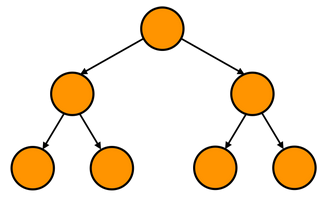Please visit, subscribe and share 10 Minutes Lectures in Computer Science

# TRUE/FALSE Quiz Questions with Answers in Data Structures and Algorithms

## Data Structures and Algorithms TRUE / FALSE Questions – Set 01

1. Binary search is always faster than linear search
(a) TRUE                                                    (b) FALSE

 Answer: FALSE Binary search is not always faster than linear search. For small number of elements (small N), say for example 20 elements, linear search is fast.

2. An objective way to compare two algorithms is by comparing their execution time irrespective of the machines
(a) TRUE                                                    (b) FALSE

 Answer: FALSE The correct way to compare two algorithms is to use big-O (asymptotic analysis).

3. When an array is passed to a function, the function receives a copy of the array (call by value)
(a) TRUE                                                    (b) FALSE

 Answer: FALSE When an array is passed as an argument to a function it is implicitly decays to a pointer that points the first element in the array. It is more costly for copying all the elements in the array to the calling function as parameters.

4. An O(log N) algorithm is slower than an O(N) algorithm
(a) TRUE                                                    (b) FALSE

 Answer: FALSE An algorithm with O(log N) time complexity is faster than O(N) algorithm. The first one is logarithmic time and the second is linear time.

### 5. Most appropriate data structure to print a list of elements in reverse order is Queue data structure

(a) TRUE                                                   (b) FALSE

Stack data structure is the most appropriate one to display a list of elements in reverse order. The concept behind stack is Last In First Out (LIFO). Hence, while reading the input elements, the first only will go to the bottom of the stack and the last is on the top. While printing, the top one will be read first and so on. As a result, we end up in displaying elements in reverse order.

### Queue uses First In First Out (FIFO), so the elements will be printed in the order they were inserted.

6. The parameter to a copy constructor must be passed by reference
(a) TRUE                                                    (b) FALSE

Pass by value would lead to infinite recursion.

### Copy Constructor

The copy constructor is a constructor which creates an object by initializing it with an object of the same class, which has been created previously.
It is very essential to pass objects as reference. If an object is passed as value to the copy Constructor then its copy constructor would call itself, to copy the actual parameter to the formal parameter. Thus an endless chain of call to the copy constructor will be initiated. This process would go on until the system run out of memory.

### 7. The largest value in a binary search tree is always stored at the right most node of the tree

(a) TRUE                                                   (b) FALSE

 Answer: TRUE An important reason that BSTs are widely used is that they allow us to keep the keys in order. A binary search tree is a binary tree with the following properties: The data stored at each node has a distinguished key which is unique in the tree and belongs to a total order. (That is, for any two non-equal keys, x,y either x < y or y < x.) The key of any node is greater than all keys occurring in its left subtree and less than all keys occurring in its right subtree.

### 8. To delete a dynamically allocated tree, the best traversal method is post-order traversal.

(a) TRUE                                                    (b) FALSE

To delete a dynamic binary tree, delete the children first, then the parent.

### Post-order traversal:

• Process the nodes in the left sub-tree with a recursive call.
• Process the nodes in the right sub-tree with a recursive call.
• Process the root.

9. Maximum number of nodes in a binary tree that has N levels is 2N.
(a) TRUE                                                    (b) FALSE

 Answer: FALSE The maximum number of nodes will be 2N-1. See the tree below with 3 levels – level 0 (root), level 1 and level 2.The given tree has maximum number of nodes which is 2N-1 = 23-1 = 7 nodes.

10. In a binary tree, every node has exactly two children.
(a) TRUE                                                    (b) FALSE

 Answer: FALSE Not exactly two children. In a binary tree, a node can have zero, one or two children.

***********